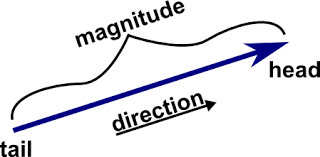## 7th GRADE SCIENCE : Article 14 : Escalar and vectorial magnitudes. Forces and directions.VECTORIAL AND ESCALAR MAGNITUDES.

* Activity 1.11  :  Vectorial and scalar magnitudes.                  ( 9/8/17   Friday )

1.11.a   Studying scalar and vectorial magnitudes  :     ( 10 points ).

Magnitude is every property that can be messured, like speed, weight, acceleration, mass, density, electrical conductivity, velocity, force, capacity, volum, etc.

- Some magnitude only need a number or quantity to be defined like the capacity and volum a bottle has, like 30 oz, or 2 liters, etc.

- Another magnitudes, like forces, velocity, etc, need both a number and a direction or vector, because they need to be determined by a direction. We must indicate in which direction, using an arrow, it goes. For instance the velocity and acceleracion a car is having need a vector, but the speed does not because speed is only a number.

1.11.b    Speed and velocity     ( 5 points ) :                                                  ( 9/11/17   Monday )

1.11.b.1  Reviewing test about vectorial and scalar magnitudes.  ( 5 points ).

Vectorial and scalar magnitudes outline picture on the whiteboard.

1.11.b.1 FILE

( 9/12/17   Tuesday )

1.11.b.2   Finishing drawings from yesterday.  Test-learning activity with the whole group about forces and vectors.    ( 5 points ).

( 9/13/17   Wednesday )

1.11.b.3Difference between speed ( scalar magnitude ) and velocity , force ( vectorial magnitudes ).

Copy the following outline in your workbook in order to check the difference between the scalar variable of speed and the vectorial one of velocity :

1.11.b.3.1  Reviewing activity 1 about drawing vectors and forces.  ( 5 points ).

Reviewing activity 2 about drawing vectors and forces.  ( 5 points ).

Speed has no direction while velocity does. For example, if I say that Im running at 10 mph, I have given you my speed. If I say that Im running 10 mph north, then I have given you my velocity.

The short answer is that velocity is the speed with a direction, while speed does not have a direction. Speed is a scalar quantity -- it is the magnitude of the velocity. Speed is measured in units of distance divided by time, e.g. miles per hour, feet per second, meters per second, etc.

( 9/14/17   Thursday )

1.11.b.3.2   Make the following drawings at your workbook and indicate the forces the cars are doing, their vectors, directions, speed and velocities vectors : ( 10 points ).

TRUE OR FALSE ABOUT FORCES AND SPEED ? :  ( 5 points each ).

1.11.c    Forces, vectorial magnitude. Parts of a force   ( 10 points ).

1.11.c.1  Therefore we can say that velocity is a vectorial magnitude and speed is a scalar magnitude. The same about forces, when a force is indicated a vector (arrow) is needed as well as the number or value, so a force is a vectorial magnitude.

( 5 points )

FORCES ARE MEASURED IN NEWTONS, THAT IS THE UNIT OF A FORCE, AND IT IS MEASURED WITH A DYNAMOMETER.

A dynamometer or "dyno" for short, is a device for measuring forces.

1.11.c.2    - Parts of a force :  ( 5 points ).

- Exercises about vectors, forces and forces vector parts :

( 9/15/17   Friday )

- 1.12    Friday activities about forces and its effects   ( 20 points ).

a.  Friday activities ( YOU CAN CHOOSE AMONG THESE POSSIBILITIES ).

National geographic magazines.

b. You can also choose to do these cross and searchwords :

Crossword

Searchword

Comentarios  Ir a formulario